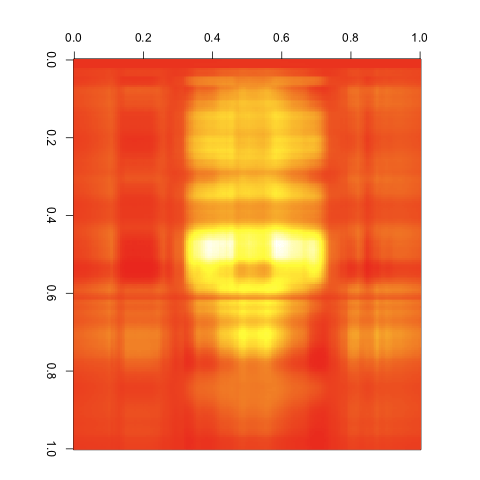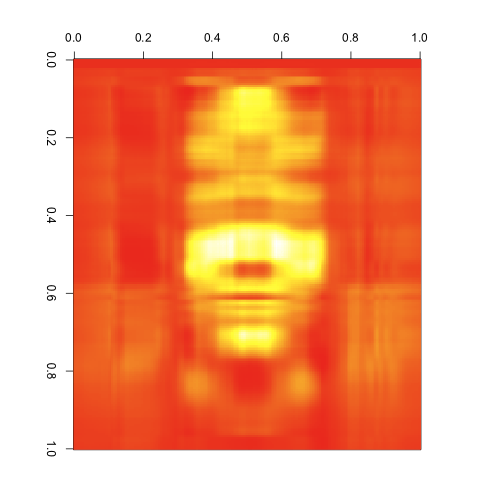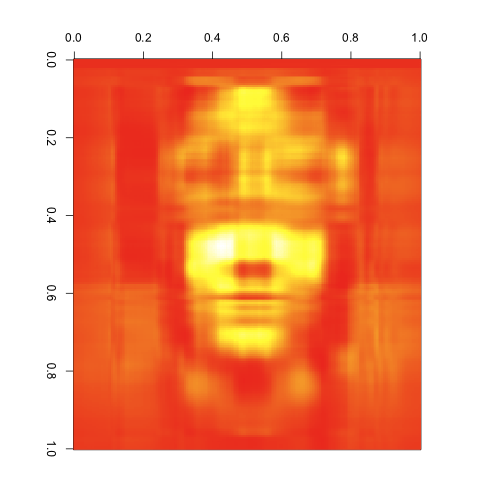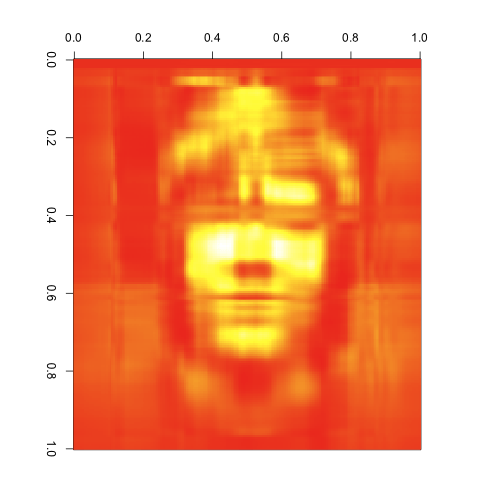[This article was first published on John Myles White: Die Sudelbücher » Statistics, and kindly contributed to R-bloggers]. (You can report issue about the content on this page here)
Want to share your content on R-bloggers? click here if you have a blog, or here if you don't.

Over the next few posts, I’m going to be reviewing the use of R to implement the most commonly used matrix techniques for image manipulation. The code will be surprisingly simple to understand, because the real magic behind these techniques lies in the mathematics that R provides an abstract interface to. To start, I’m going to review the singular value decomposition of a matrix, also known as the SVD.

If you’d like to understand the magic behind the scenes here, you can read about the mathematical definition of the SVD on Wikipedia or you can watch Gilbert Strang’s lectures. If your command of linear algebra isn’t great, I suspect that the mathematical details of the SVD will be totally opaque to you.

Fortunately, the details of the underlying mathematics aren’t at all necessary for appreciating the SVD’s usefulness for manipulating images: it is arguably the best way to compress matrices into smaller representations that contain the essential information from the original matrices. The easiest way to understand this is to see it in action, so I’m going to show how the SVD allows for any degree of compression of an image represented as a real-valued matrix.

Obviously, the first thing we have to do is to represent our example image as a matrix with real-valued entries. To do this, we’ll need to use a simple technique for loading an image into R that I found on the Quantitative Ecology blog. The first thing to do is use ImageMagick to transform a TIFF file into a PPM file:

 ```1 2 3 ``` ```#!/bin/bash   convert face.tiff image.ppm```

Then we can use the “pixmap” package from CRAN to read the PPM image into R as an image object containing three matrices: one for the red components, one for the green components, and one for the blue components. The exact code I used was this:

 ```1 2 3 4 5 6 7 ``` ```library('pixmap')   image <- read.pnm('image.ppm')   red.matrix <- matrix([email protected], nrow = [email protected], ncol = [email protected]) green.matrix <- matrix([email protected], nrow = [email protected], ncol = [email protected]) blue.matrix <- matrix([email protected], nrow = [email protected], ncol = [email protected])```

Before we go any further, we should look at the image we’ll be playing with. Rather than work with the composite of the three color matrices, I found it easier to work with only a single color. For this specific image, I found that the green matrix looked best, so I’ll use only that. At the risk of seeming self-indulgent, I’m using my own Gravatar icon as an example image because faces are particularly easy to recognize under the strange color scheme I’ll be using, which I chose because it really simplifies the visualization code.

To visualize the matrix as an image, I discovered two very helpful graphical functions, `image` and `heat.colors`. `image` depicts the value of a matrix using a color scheme you specify; `heat.colors` produces a set of colors that could be used in a heat map with a granularity you specify as the first argument. Using these functions, we can see our raw input matrix:

 ```1 ``` `image(green.matrix, col = heat.colors(255))`

The images generated by `image` are at an unfortunate angle for viewing, so I edited them post hoc with a single 90º clockwise rotation in Preview to give this sort of image:If you want to play with the original PPM image file, you can get it here.

Clearly, we’ve got a recognizable face here. Feeling good about that, we can start to play with the SVD of this matrix. The R function to generate the SVD of a matrix is simply `svd`:

 ```1 ``` `green.matrix.svd <- svd(green.matrix)`

`svd` returns a list with three entries: `d`, `u` and `v`. `d` is a vector of the singular values ordered by decreasing size; `u` and `v` are matrices that are combined with a diagonal matrix form of `d` to give the original input matrix. To simplify things, I’ll set up variables to contain the value of these elements of the SVD output list:

 ```1 2 3 ``` ```d <- green.matrix.svd\$d u <- green.matrix.svd\$u v <- green.matrix.svd\$v```

We can check that the SVD works by multiplying the relevant matrices:

 ```1 2 3 4 ``` ```green.matrix.reconstruction <- u %*% diag(d) %*% t(v)   mean(green.matrix - green.matrix.reconstruction) #  -5.184204e-16```

If this is the SVD by itself, it’s not clear that it’s useful computationally: we’ve just rewritten one matrix as the product of three matrices and accumulated a small bit of error along the way. The real utility of the SVD lies in the singular values: they represent, in decreasing order, the most important information about the original matrix.

To see this, you can shrink the input matrices and produce a compressed form of the matrix. For instance, my original matrix is a 212 by 201 image. Using the SVD, we can represent it as the product of a 212 by 2 matrix, a 2 by 2 matrix, and a 2 by 201 matrix. This massively reduces the amount of information we’re storing, but you can already see the outline of our input image in the results:

 ```1 2 3 ``` ```green.matrix.compressed <- u[,1:i] %*% diag(d[1:i]) %*% t(v[,1:i])   image(green.matrix.compressed, col = heat.colors(255))```To get a feel for how this works, we can iterate over the number of singular values we include in our compressed form:

 ```1 2 3 4 5 6 7 ``` ```for (i in c(3, 4, 5, 10, 20, 30)) { green.matrix.compressed <- u[,1:i] %*% diag(d[1:i]) %*% t(v[,1:i]) png(paste('images/Green Image SVD Compressed ', i, '.png', sep = '')) image(green.matrix.compressed, col = heat.colors(255)) dev.off() }```

This produces the following images:

#### 3 Singular Values#### 4 Singular Values#### 5 Singular Values#### 10 Singular Values#### 20 Singular Values#### 30 Singular ValuesAs you can see, the images get better quite quickly. Indeed, this last image looks great, despite the large reduction in the number of entries we’re keeping from the original matrix. I’d say that’s a pretty compelling case for the value of the SVD. It would be easy to combine the compressed matrices for red, green and blue to get a compressed version of our input image using its original color scheme. I’ll leave that as an exercise for anyone interested in playing with this technique on their own.

Of course, the SVD has tons of other uses, but this simple hack for image compression struck me as pretty interesting, as well as being remarkably simple to implement in R.